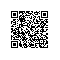# 关于浮点数和字符串转换的函数示例

1、float  stof(char *);                    //十进制字符串 --> float　　（如："-1.0"　-->  0xFF800000）
2、float  fadd(float, float);
3、float  fsub(float, float);
4、float  fmul(float, float);
5、float  fdiv(float, float);
6、void  ftos(char *, float);           //float --> 十进制字符串(如：0x40000000 --> "2.0")

Code:
1. bool IsNumber(char c)
2. {
3.     if('0'>c) return false;
4.     if('9'<c) return false;
5.     return true;
6. }
7. double stof(char* szFloatString)
8. {
9.     double fRet=0.0;    //试了一下，不能用float，精度太低了，我改用double
10.     double fRetLittleNumber=0.0;
11.     int nStringLength=strlen(szFloatString);
12.     int i=0;
13.     if(0>=nStringLength)
14.         goto stof_End_Process_Error;
15.     char cTarget=0;
16.
17.     i=0;                //这是在找整数部分
18.     //整数部分顺找，找到一个数字，基数*10+这个数字，就是把数字用10进制拼接到基数中
19.     while(1)
20.     {
21.         cTarget=*(szFloatString+i);
22.         if('.'==cTarget)
23.             goto stof_Get_Little_Number;
24.         if('\0'==cTarget)
25.             goto stof_End_Process;
26.         if(!IsNumber(cTarget))
27.             goto stof_End_Process_Error;
28.         cTarget-='0';
29.         fRet*=10.0;     //先*10
30.         fRet+=cTarget;  //再+，原来是1，新数字是2，1*10+2=12
31.         i++;
32.     }
33. stof_Get_Little_Number:     //这是在找小数部分
34.     //小数部分，从右向左逆找
35.     //找到新数字，基数+新数字/10，这是把新数字拼接到左边
36.     i=nStringLength-1;
37.     while(1)
38.     {
39.         cTarget=*(szFloatString+i);
40.         if('.'==cTarget)
41.             goto stof_I_Get_It;
42.         if(!IsNumber(cTarget))
43.             goto stof_End_Process_Error;
44.         cTarget-='0';
45.         fRetLittleNumber+=cTarget;  //先+新数字
46.         fRetLittleNumber/=10.0;     //再/10，0+1/10=0.1，0.1+2/10=0.21，明白没有？
47.         i--;
48.     }
49. stof_I_Get_It:          //找到了，拼接数字
50.     fRet+=fRetLittleNumber;
51.     goto stof_End_Process;
52. stof_End_Process_Error: //这是出错了
53.     printf("\"%s\" is not float!\n",szFloatString);
54.     fRet=0.0;
55. stof_End_Process:       //这是返回点
56.     return fRet;
57. }
58. void Test_stof(void)
59. {
60.     char* pStr="";
61.     pStr="123";
62.     printf("\"%s\"=%f\n",pStr,stof(pStr));
63.     pStr="123.321";
64.     printf("\"%s\"=%f\n",pStr,stof(pStr));
65.     pStr="1234567890.0987654321";
66.     printf("\"%s\"=%f\n",pStr,stof(pStr));
67. }

Code:
1. "123"=123.000000
2. "123.321"=123.321000
3. "1234567890.0987654321"=1234567890.098765

C语言准确地讲，不是一门太适合数学计算的语言，它浮点精度始终有限，如果要做纯正的数学计算，建议用Fortran等专业的数学计算语言。

sprintf(szBuffer,"%f",fResult);

Code:
1. int SafePrintf(char* szBuf,int nMaxLength,char *szFormat, ...)
2. {
3.     int nListCount=0;
4.     va_list pArgList;
5.
6.     if (!szBuf) goto SafePrintf_END_PROCESS;
7.     va_start (pArgList,szFormat);
8.     nListCount+=Linux_Win_vsnprintf(szBuf+nListCount,
9.         nMaxLength-nListCount,szFormat,pArgList);
10.     va_end(pArgList);
11.     if(nListCount>(nMaxLength-1)) nListCount=nMaxLength-1;
12.     *(szBuf+nListCount)='\0';
13.
14. SafePrintf_END_PROCESS:
15.     return nListCount;
16. }
简单说，就是给出字符串缓冲区，还要给出一个缓冲区大小，我的函数保证不会打出去溢出，还有，就是不管缓冲区够不够，总之保证你最后得到一个'\0'的结束符。使用钉钉扫一扫加入圈子
+ 订阅